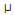# Electronics - Capacitors - Discussion

### Discussion :: Capacitors - General Questions (Q.No.21)

21.

If a current of 40 mA flows through a series circuit consisting of a 0.4F capacitor and a resistor in series with a 4 kHz, 40 Vac source, what is the total circuit impedance?

 [A]. 10[B]. 100[C]. 1 k[D]. 1 MExplanation:

No answer description available for this question.

 M.V.Krishna said: (Sep 3, 2011) V=I*R; V=40,I=40mA; R=40V/40mA; R=1kohm

 Rita said: (Sep 12, 2011) What about capacitive reactance? Total impedance is required which also include Xc.

 Ravi Jumwal said: (Oct 4, 2011) This is the total current flowing through the circuit so where the matter of Xc come from.

 Sobia said: (Apr 16, 2012) Reactance if we calculate Xc=1/(2*pi*f*c) is 99.47 which is very low as compared to R which is 1k by above formula explained by Krishna so we ignore 99.91 and call total resistance 1k

 Divik said: (May 19, 2012) The solution of question also states that with such alternating high frequency the capacitive reactance becomes negligible as it also the fundamental property of capacitor.

 Qummar said: (Aug 1, 2012) We get Xc = 99.47 and R = 1k and we know that total impedance is sqrt (R*R+Xc*Xc) = 1.004k.

 Mukesh said: (Nov 13, 2012) As Xc=99.47; Now, (Vt)^2=I^2[R^2 + Xc^2]; (40)^2=(40*10^-3)^2[R^2 + (99.47)^2]; R=995. Approximately 1k.

 Arun said: (Oct 24, 2013) Xc = 99.52 OHM. Vc = I*Xc = 3.98 V. Vr = SQRT (Vs^2-Vc^2) = 39.8 V. Z= SQRT (R^2+ Xc^2) = 999.99 OHM ~ 1 KILO OHM.

 Al Ongsuco said: (Nov 11, 2014) How did you get the frequency?

 Shubham said: (Jan 30, 2015) What is total circuit impedance?

 Ameya Pagare said: (Jul 18, 2019) The value of XC is given by the passive HPF formula for Xc. Xc=1/2*3.14*R*C. Xc= 99.52 ohm.

 Kiran V said: (Sep 19, 2020) Given: I = 40 m A; C = 0.4μF; f = 4 kHz; V = 40 Vac; ZT =? Z = Vac /I. Z = 40 / 40 m A = 1 * 10^3. Z = 1 k Ω.